# 摘要：

## 1.GB原理概述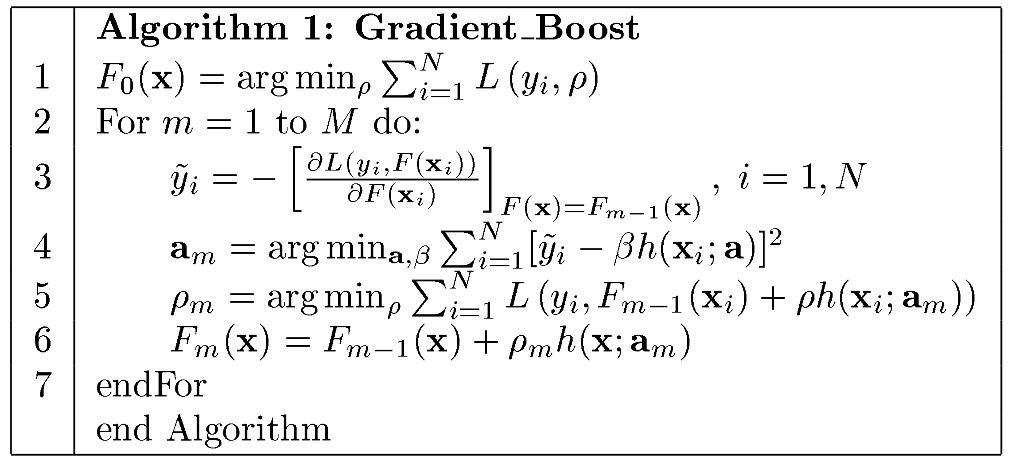$L\left({y}_{i},F\left({x}_{i}\right)\right)=\left(\frac{1}{2}\right)\ast \left({y}_{i}-F\left({x}_{i}\right){\right)}^{2}$$\large L(y_i,F(x_i))=\left(\frac{1}{2}\right)*(y_i-F(x_i))^2$,那么其负梯度值为：$-\left[\frac{\mathrm{\partial }L\left({y}_{i},F\left({\mathbf{x}}_{i}\right)\right)}{\mathrm{\partial }F\left({\mathbf{x}}_{i}\right)}\right]=\left({y}_{i}-F\left({x}_{i}\right)\right)$$\large -\left[\frac{\partial L(y_i,F(\mathbf{x}_i))}{\partial F(\mathbf{x}_i)}\right]=(y_i-F(x_i))$，再带入当前模型的值$F\left(x\right)={F}_{m-1}\left(x\right)$$\large {F(x)=F_{m-1}(x)}$

$\stackrel{~}{{y}_{i}}=-{\left[\frac{\mathrm{\partial }L\left({y}_{i},F\left({\mathbf{x}}_{i}\right)\right)}{\mathrm{\partial }F\left({\mathbf{x}}_{i}\right)}\right]}_{F\left(x\right)={F}_{m-1}\left(x\right)}=\left({y}_{i}-{F}_{m-1}\left({x}_{i}\right)\right)$$\large \tilde{y_i}=-\left[\frac{\partial L(y_i,F(\mathbf{x}_i))}{\partial F(\mathbf{x}_i)}\right]_{F(x)=F_{m-1}(x)}=(y_i-F_{m-1}(x_i))$

$L\left({y}_{i},F\left({x}_{i}\right)\right)=|{y}_{i}-F\left({x}_{i}\right)|$$\large L(y_i,F(x_i))=\left|y_i-F(x_i)\right|$，其梯度值为：
$\stackrel{~}{{y}_{i}}=-{\left[\frac{\mathrm{\partial }L\left({y}_{i},F\left({\mathbf{x}}_{i}\right)\right)}{\mathrm{\partial }F\left({\mathbf{x}}_{i}\right)}\right]}_{F\left(x\right)={F}_{m-1}\left(x\right)}=sign\left({y}_{i}-{F}_{m-1}\left({x}_{i}\right)\right)$$\large \tilde{y_i}=-\left[\frac{\partial L(y_i,F(\mathbf{x}_i))}{\partial F(\mathbf{x}_i)}\right]_{F(x)=F_{m-1}(x)}=sign\left(y_i-F_{m-1}(x_i)\right)$

$L\left({y}_{i},F\left({x}_{i}\right)\right)={y}_{i}log\left({p}_{i}\right)+\left(1-{y}_{i}\right)log\left(1-{p}_{i}\right)$$\large L\left(y_i,F(x_i)\right)=y_ilog(p_i)+(1-y_i)log(1-p_i)$

$\stackrel{~}{{y}_{i}}=-{\left[\frac{\mathrm{\partial }L\left({y}_{i},F\left({\mathbf{x}}_{i}\right)\right)}{\mathrm{\partial }F\left({\mathbf{x}}_{i}\right)}\right]}_{F\left(x\right)={F}_{m-1}\left(x\right)}={y}_{i}-\frac{1}{1+{e}^{-{F}_{m-1}\left({x}_{i}\right)}}$$\large \tilde{y_i}=-\left[\frac{\partial L(y_i,F(\mathbf{x}_i))}{\partial F(\mathbf{x}_i)}\right]_{F(x)=F_{m-1}(x)}=y_i-\frac{1}{1+e^{-F_{m-1}(x_i)}}$（这个简单推导过程在下一篇文章有，以及多分类任务采用的loss-function)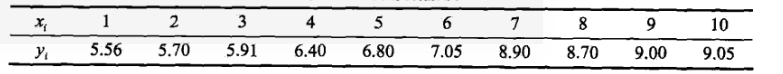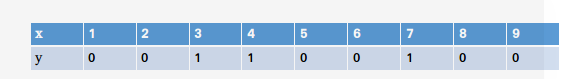${F}_{0}\left(x\right)=\left(\frac{1}{2}\right)\ast log\left(\frac{\sum {y}_{i}}{\sum \left(1-{y}_{i}\right)}\right)=\left(\frac{1}{2}\right)\ast log\left(\frac{3}{7}\right)$$\large F_0(x)=\left(\frac{1}{2}\right)*log\left(\frac{\sum{y_i}}{\sum{(1-y_i)}}\right)=\left(\frac{1}{2}\right)*log\left(\frac{3}{7}\right)$

$L\left({y}_{i},F\left({x}_{i}\right)\right)={e}^{-yF\left({x}_{i}\right)}$$\large L\left(y_i,F(x_i)\right)=e^{-yF(x_i)}$，其负梯度大家可以自己求求，后面有汇总表给大家参考。

## 2.GBDT原理-2

$L\left({y}_{i},F\left({x}_{i}\right)\right)=\left(\frac{1}{2}\right)\ast \left({y}_{i}-F\left({x}_{i}\right){\right)}^{2}$$\large L(y_i,F(x_i))=\left(\frac{1}{2}\right)*(y_i-F(x_i))^2$

${\gamma }_{jm}=av{e}_{{x}_{i}\in {R}_{jm}}\stackrel{~}{{y}_{i}}$$\large \gamma_{jm}=ave_{{x_i} \in R_{jm}}\tilde{y_i}$$\stackrel{~}{{y}_{i}}$$\tilde{y_i}$为梯度值。

${\gamma }_{jm}=media{n}_{{x}_{i}\in {R}_{jm}}\left({y}_{i}-{F}_{m-1}\left({x}_{i}\right)\right)$$\large \gamma_{jm}=median_{{x_i} \in R_{jm}}\left({y_i-F_{m-1}(x_i)}\right)$

${\gamma }_{jm}=\frac{\sum _{i=1}^{N}\stackrel{~}{{y}_{i}}}{\sum _{i=1}^{N}\left({y}_{i}-\stackrel{~}{{y}_{i}}\right)\ast \left(1-{y}_{i}+\stackrel{~}{{y}_{i}}\right)}$$\large \gamma_{jm}=\frac{\sum_{i=1}^{N}\tilde{y_i}}{\sum_{i=1}^{N}(y_i-\tilde{y_i})*(1-y_i+\tilde{y_i})}$

${\gamma }_{jm}=\frac{\sum _{i=1}^{N}\left(2{y}_{i}-1\right){e}^{\left(-\left(2{y}_{i}-1\right){F}_{m-1}\left({x}_{i}\right)\right)}}{\sum _{i=1}^{N}{e}^{\left(-\left(2{y}_{i}-1\right){F}_{m-1}\left({x}_{i}\right)\right)}}$$\large \gamma_{jm}=\frac{\sum_{i=1}^{N}(2y_i-1)e^{\left(-(2y_i-1)F_{m-1}(x_i)\right)}}{\sum_{i=1}^{N}e^{\left(-(2y_i-1)F_{m-1}(x_i)\right)}}$

## 3.GBDT实践以及Sklearn源码分析

${x}_{i}$$x_i$ 1 2 3 4 5 6 7 8 9 10
${\stackrel{~}{y}}_{i}$$\tilde{y}_i$ 5.56 5.7 5.91 6.4 6.8 7.05 8.9 8.7 9. 9.05

1. 选择MSE做为建树的分裂准则
2. 选择MSE作为误差函数
3. 树的深度设置为1

$\stackrel{~}{{y}_{i}}=-{\left[\frac{\mathrm{\partial }L\left({y}_{i},F\left({\mathbf{x}}_{i}\right)\right)}{\mathrm{\partial }F\left({\mathbf{x}}_{i}\right)}\right]}_{F\left(x\right)={F}_{m-1}\left(x\right)}=\left({y}_{i}-{F}_{m-1}\left({x}_{i}\right)\right)$$\tilde{y_i}=-\left[\frac{\partial L(y_i,F(\mathbf{x}_i))}{\partial F(\mathbf{x}_i)}\right]_{F(x)=F_{m-1}(x)}=(y_i-F_{m-1}(x_i))$

${x}_{i}$$x_i$ 1 2 3 4 5 6 7 8 9 10
${\stackrel{~}{y}}_{i}$$\tilde{y}_i$ -1.747 -1.607 -1.397 -0.907 -0.507 -0.257 1.593 1.393 1.693 1.743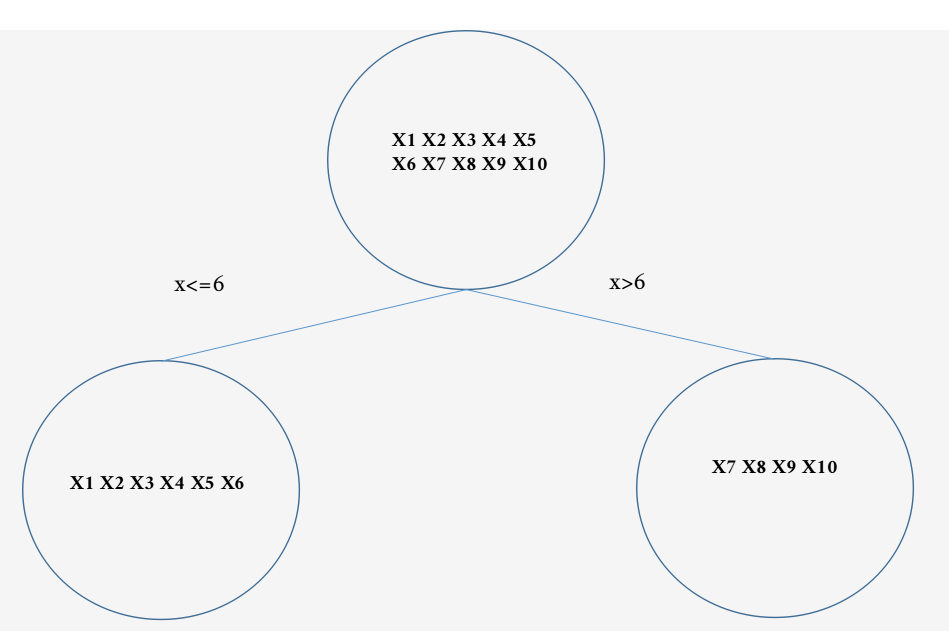${R}_{11}为{x}_{i}<=6$$R_{11}为x_i<=6$${R}_{21}为{x}_{i}>6$$R_{21}为x_i>6$
${\gamma }_{11}=\frac{\left({\stackrel{~}{y}}_{1}+{\stackrel{~}{y}}_{2}+{\stackrel{~}{y}}_{3}+{\stackrel{~}{y}}_{4}+{\stackrel{~}{y}}_{5}+{\stackrel{~}{y}}_{6}\right)}{6}=-1.0703$$\gamma_{11}=\frac{\left(\tilde{y}_1+\tilde{y}_2+\tilde{y}_3+\tilde{y}_4+\tilde{y}_5+\tilde{y}_6\right)}{6}=-1.0703$
${\gamma }_{21}=\frac{\left({\stackrel{~}{y}}_{7}+{\stackrel{~}{y}}_{8}+{\stackrel{~}{y}}_{9}+{\stackrel{~}{y}}_{10}\right)}{4}=1.6055$$\gamma_{21}=\frac{\left(\tilde{y}_7+\tilde{y}_8+\tilde{y}_9+\tilde{y}_{10}\right)}{4}=1.6055$

${F}_{1}\left({x}_{1}\right)={F}_{0}\left({x}_{1}\right)+\sum _{j=1}^{2}{\gamma }_{j1}I\left({x}_{1}\in {R}_{j1}\right)=7.307-1.0703=6.2367$$F_1(x_1)=F_0(x_1)+\sum_{j=1}^2\gamma_{j1}I(x_1\in R_{j1})=7.307-1.0703=6.2367$

${F}_{m}\left(x\right)={F}_{m-1}\left(x\right)+\eta \ast \sum _{j=1}^{J}{\gamma }_{jm}I\left(x\in {R}_{jm}\right)$$F_m(x)=F_{m-1}(x)+\eta*\sum_{j=1}^J\gamma_{jm}I(x \in R_{jm})$$\eta$$\eta$为学习率。所以，当$\eta =0.1$$\eta=0.1$时，上面的计算结果变为：
${F}_{1}\left({x}_{1}\right)={F}_{0}\left({x}_{1}\right)+0.1\ast \sum _{j=1}^{2}{\gamma }_{j1}I\left({x}_{1}\in {R}_{j1}\right)=7.307-0.1\ast 1.0703=7.1997$$F_1(x_1)=F_0(x_1)+0.1*\sum_{j=1}^2\gamma_{j1}I(x_1\in R_{j1})=7.307-0.1*1.0703=7.1997$

$\stackrel{~}{{y}_{1}}=-{\left[\frac{\mathrm{\partial }L\left({y}_{i},F\left({\mathbf{x}}_{i}\right)\right)}{\mathrm{\partial }F\left({\mathbf{x}}_{i}\right)}\right]}_{F\left(x\right)={F}_{m-1}\left(x\right)}=\left({y}_{1}-{F}_{1}\left({x}_{1}\right)\right)=\left(5.56-7.19996\right)=-1.63996667$$\tilde{y_1}=-\left[\frac{\partial L(y_i,F(\mathbf{x}_i))}{\partial F(\mathbf{x}_i)}\right]_{F(x)=F_{m-1}(x)}=(y_1-F_{1}(x_1))=(5.56-7.19996)=-1.63996667$

${x}_{i}$$x_i$ 1 2 3 4 5 6 7 8 9 10
${\stackrel{~}{y}}_{i}$$\tilde{y}_i$ -1.63996667 -1.49996667 -1.28996667 -0.79996667 -0.39996667 -0.14996667 1.43245 1.23245 1.53245 1.58245

${\gamma }_{12}=-0.9633$$\gamma_{12}=-0.9633$
${\gamma }_{22}=1.44495$$\gamma_{22}=1.44495$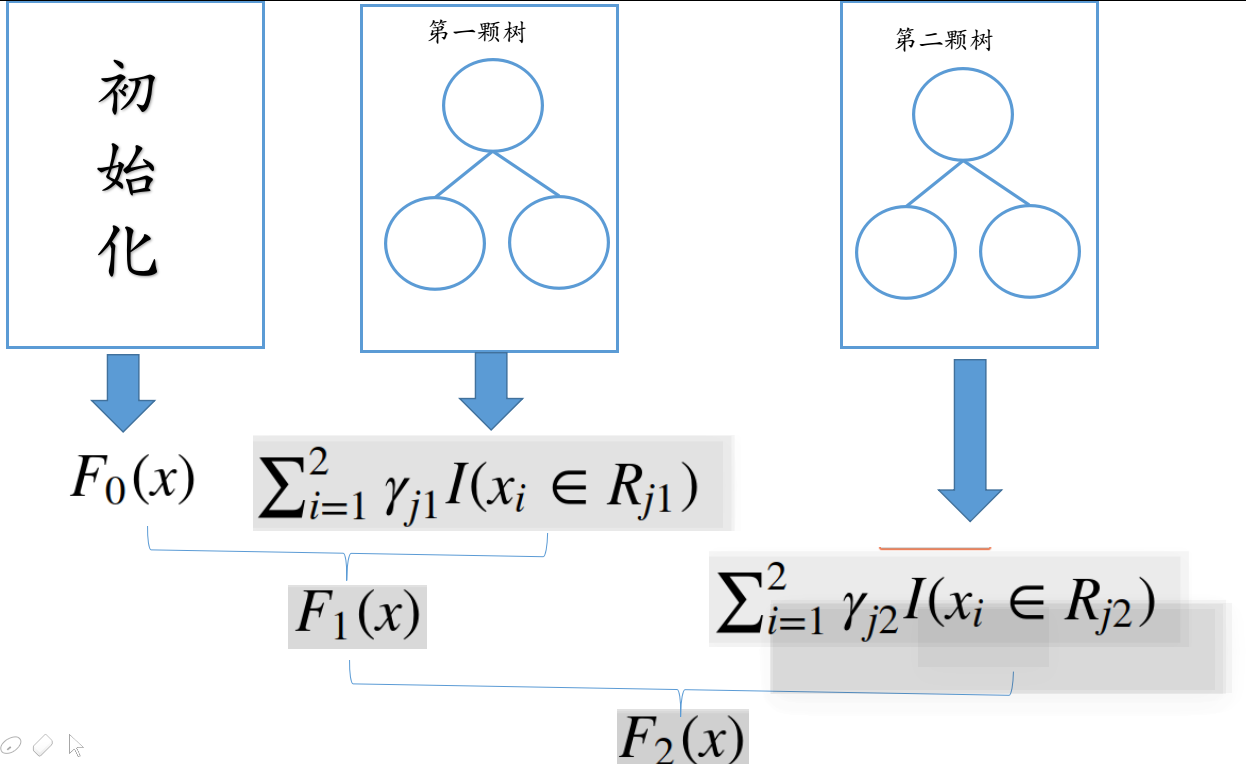## Sklearn源码分析

Sklearn里面，当loss function选择mse时，计算负梯度值、计算叶子节点的值是在一个叫LeastSquaresError的类里面实现的。

class LeastSquaresError(RegressionLossFunction):
"""Loss function for least squares (LS) estimation.
Terminal regions need not to be updated for least squares. """
def init_estimator(self):
return MeanEstimator()

def __call__(self, y, pred, sample_weight=None):
if sample_weight is None:
return np.mean((y - pred.ravel()) ** 2.0)
else:
return (1.0 / sample_weight.sum() *
np.sum(sample_weight * ((y - pred.ravel()) ** 2.0)))

return y - pred.ravel()

def update_terminal_regions(self, tree, X, y, residual, y_pred,
learning_rate=1.0, k=0):
"""Least squares does not need to update terminal regions.

But it has to update the predictions.
"""
# update predictions
print ("树节点值",tree.value)
y_pred[:, k] += learning_rate * tree.predict(X).ravel()
def _update_terminal_region(self, tree, terminal_regions, leaf, X, y,
residual, pred, sample_weight):
pass


    def negative_gradient(self, y, pred, **kargs):
return y - pred.ravel()

class MeanEstimator(object):
"""An estimator predicting the mean of the training targets."""
def fit(self, X, y, sample_weight=None):
if sample_weight is None:
self.mean = np.mean(y)
else:
self.mean = np.average(y, weights=sample_weight)

def predict(self, X):
check_is_fitted(self, 'mean')
y = np.empty((X.shape, 1), dtype=np.float64)
y.fill(self.mean)
return y


    def fit(self, X, y, sample_weight=None):
if sample_weight is None:
self.mean = np.mean(y)
else:
self.mean = np.average(y, weights=sample_weight)


def update_terminal_regions(self, tree, X, y, residual, y_pred,
learning_rate=1.0, k=0):
"""Least squares does not need to update terminal regions.

But it has to update the predictions.
"""
# update predictions
y_pred[:, k] += learning_rate * tree.predict(X).ravel()

            # induce regression tree on residuals
tree = DecisionTreeRegressor(
criterion=self.criterion,
splitter='best',
max_depth=self.max_depth,
min_samples_split=self.min_samples_split,
min_samples_leaf=self.min_samples_leaf,
min_weight_fraction_leaf=self.min_weight_fraction_leaf,
min_impurity_decrease=self.min_impurity_decrease,
min_impurity_split=self.min_impurity_split,
max_features=self.max_features,
max_leaf_nodes=self.max_leaf_nodes,
random_state=random_state,
presort=self.presort)